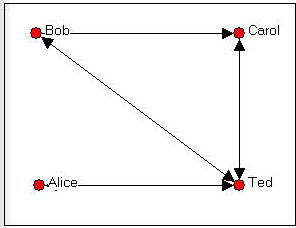$$\newcommand{\vecs}{\overset { \rightharpoonup} {\mathbf{#1}} }$$ $$\newcommand{\vecd}{\overset{-\!-\!\rightharpoonup}{\vphantom{a}\smash {#1}}}$$$$\newcommand{\id}{\mathrm{id}}$$ $$\newcommand{\Span}{\mathrm{span}}$$ $$\newcommand{\kernel}{\mathrm{null}\,}$$ $$\newcommand{\range}{\mathrm{range}\,}$$ $$\newcommand{\RealPart}{\mathrm{Re}}$$ $$\newcommand{\ImaginaryPart}{\mathrm{Im}}$$ $$\newcommand{\Argument}{\mathrm{Arg}}$$ $$\newcommand{\norm}{\| #1 \|}$$ $$\newcommand{\inner}{\langle #1, #2 \rangle}$$ $$\newcommand{\Span}{\mathrm{span}}$$ $$\newcommand{\id}{\mathrm{id}}$$ $$\newcommand{\Span}{\mathrm{span}}$$ $$\newcommand{\kernel}{\mathrm{null}\,}$$ $$\newcommand{\range}{\mathrm{range}\,}$$ $$\newcommand{\RealPart}{\mathrm{Re}}$$ $$\newcommand{\ImaginaryPart}{\mathrm{Im}}$$ $$\newcommand{\Argument}{\mathrm{Arg}}$$ $$\newcommand{\norm}{\| #1 \|}$$ $$\newcommand{\inner}{\langle #1, #2 \rangle}$$ $$\newcommand{\Span}{\mathrm{span}}$$$$\newcommand{\AA}{\unicode[.8,0]{x212B}}$$

The most common form of matrix in social network analysis is a very simple square matrix with as many rows and columns as there are actors in our data set. The "elements" or scores in the cells of the matrix record information about the ties between each pair of actors.

The simplest and most common matrix is binary. That is, if a tie is present, a one is entered in a cell; if there is no tie, a zero is entered. This kind of a matrix is the starting point for almost all network analysis, and is called an "adjacency matrix" because it represents who is next to, or adjacent to whom in the "social space" mapped by the relations that we have measured.

An adjacency matrix may be "symmetric" or "asymmetric." Social distance can be either symmetric or asymmetric. If Bob and Carol are "friends" they share a "bonded tie" and the entry in the Xi,j cell will be the same as the entry in the Xj,i cell.

But social distance can be a funny (non-Euclidean) thing. Bob may feel close to Carol, but Carol may not feel the same way about Bob. In this case, the element showing Bob's relationship to Carol would be scored "1," while the element showing Carol's relation to Bob would be scored "0." That is, in an "asymmetric" matrix, Xi,j is not necessarily equal to Xj,i.

By convention, in a directed (i.e. asymmetric) matrix, the sender of a tie is the row and the target of the tie is the column. Let's look at a simple example. The directed graph of friendship choices among Bob, Carol, Ted, and Alice is shown in figure 5.4.Figure 5.4. Bob, Carol, Ted, and Alice

We can since the ties are measured at the nominal level (that is, the data are binary choice data), we can represent the same information in a matrix that looks like:

 Bob Carol Ted Alice Bob --- 1 1 0 Carol 0 --- 1 0 Ted 1 1 --- 1 Alice 0 0 1 ---

Figure 5.5. Asymmetric adjacency matrix of the graph shown in Figure 5.4.

Remember that the rows represent the source of directed ties, and the columns the targets; Bob chooses Carol here, but Carol does not choose Bob. This is an example of an "asymmetric" matrix that represents directed ties (ties that go from a source to a receiver). That is, the element i,j does not necessarily equal the element j,i. If the ties that we were representing in our matrix were "bonded-ties" (for example, ties representing the relation "is a business partner of" or "co-occurrence or co-presence," (e.g. where ties represent a relation like: "serves on the same board of directors as") the matrix would necessarily be symmetric; that is element i,j would be equal to element j,i.

Binary choice data are usually represented with zeros and ones, indicating the presence or absence of each logically possible relationship between pairs of actors.

Signed graphs are represented in matrix form (usually) with -1, 0, and +1 to indicate negative relations, no or neutral relations, and positive relations. "Signed" graphs are actually a specialized version of an ordinal relation.

When ties are measured at the ordinal or interval level, the numeric magnitude of the measured tie is entered as the element of the matrix. As we discussed earlier, other forms of data are possible (multi-category nominal, ordinal with more than three ranks, full-rank order nominal). These other forms, however, are rarely used in sociological studies, and we won't give them very much attention.

In representing social network data as matrices, the question always arises: what do I do with the elements of the matrix where i = j? That is, for example, does Bob regard himself as a close friend of Bob? This part of the matrix is called the main diagonal. Sometimes the value of the main diagonal is meaningless, and it is ignored (and left blank or filled with zeros or ones). Sometimes, however, the main diagonal can be very important, and can take on meaningful values. This is particularly true when the rows and columns of our matrix are "super-nodes" or "blocks." More on that in a minute.

It is often convenient to refer to certain parts of a matrix using shorthand terminology. If I take all of the elements of a row (e.g. who Bob chose as friends: ---,1,1,0) I am examining the "row vector" for Bob. If I look only at who chose Bob as a friend (the first column, or ---,0,1,0), I am examining the "column vector" for Bob. It is sometimes useful to perform certain operations on row or column vectors. For example, if I summed the elements of the column vectors in this example, I would be measuring how "popular" each node was (in terms of how often they were the target of a directed friendship tie). So a "vector" can be an entire matrix (1 x ... or ...x 1), or a part of a larger matrix.

This page titled 5.2: The "adjacency" matrix is shared under a not declared license and was authored, remixed, and/or curated by Robert Hanneman & Mark Riddle.